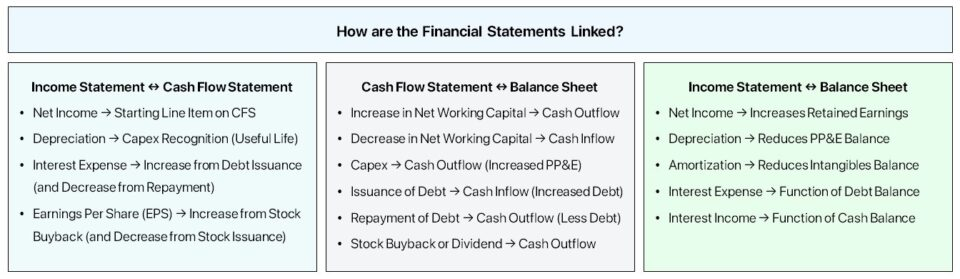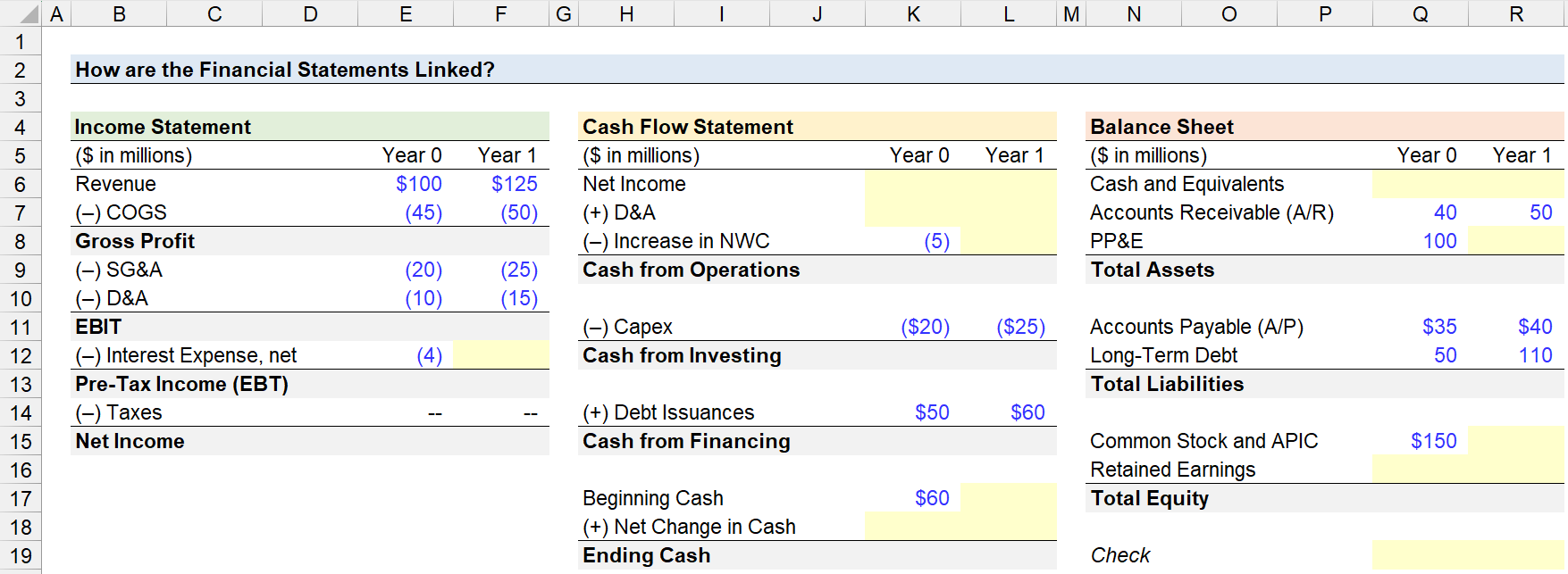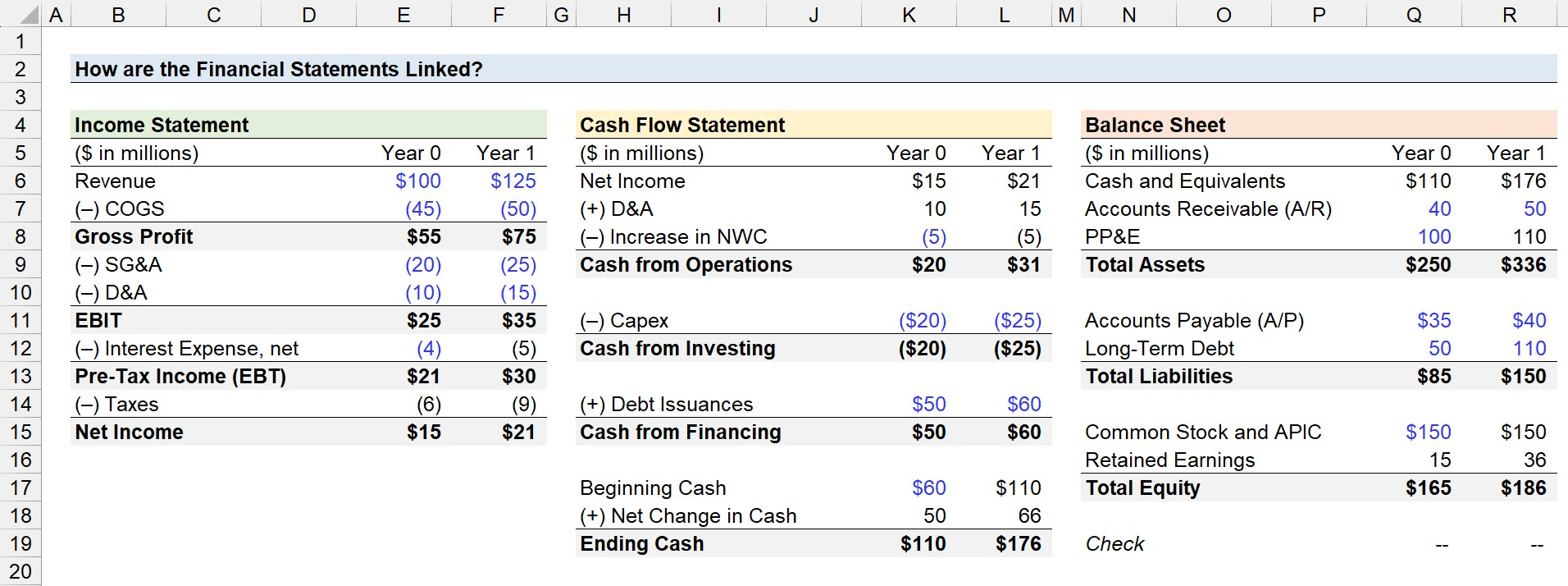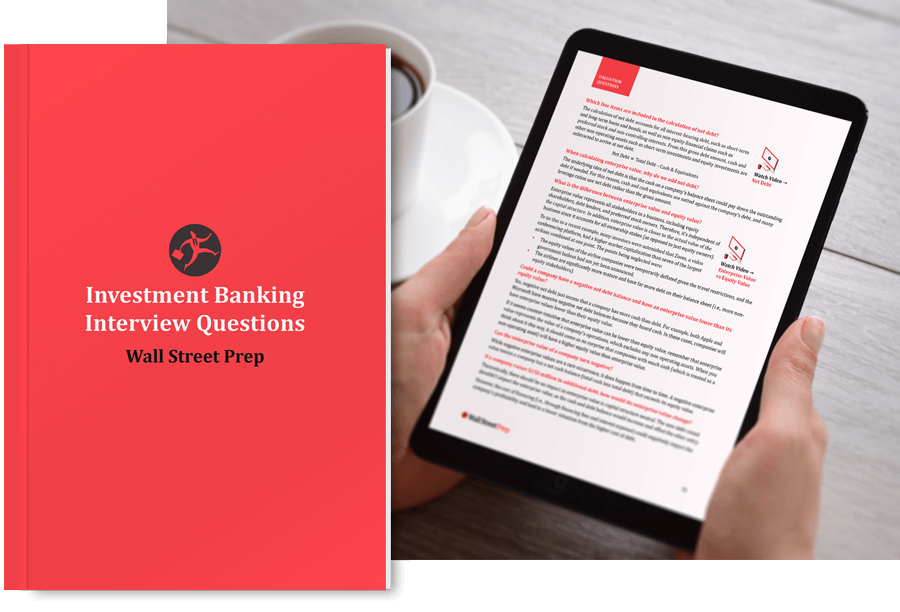Welcome to Wall Street Prep! Use code at checkout for 15% off.# How are the Financial Statements Linked?

Guide to Understanding How to Answer the, "How are the Three Financial Statements Linked?" Interview Question (2023 Update)The three core financial statements – the income statement, balance sheet, and cash flow statement – are closely intertwined under accrual accounting.

To answer the common accounting interview question accurately, the principles of accrual accounting (U.S. GAAP) must be understood, including a familiarity with the underlying mechanisms of an integrated 3-statement model.

Otherwise, your response to the interview question will be sub-par and based on mere memorization, rather than a real understanding of the interconnections between the three financial statements.

Financial Statement Description
Income Statement (I/S)
• The income statement, or profit and loss statement (P&L), shows a company’s accrual-based profitability over a specified time period.
• The standard filing dates are on a quarterly and annual basis in the U.S.
• The starting line item is revenue (the “top line”) and after deducting various costs and expenses, the final line item is net income (the “bottom line”).
Balance Sheet (B/S)
• The balance sheet is a “snapshot” of a company’s assets, liabilities, and shareholders’ equity at a specific point in time, such as the end of a quarter or fiscal year.
• The assets side of the balance sheet represents the “resources” owned by the company with positive economic utility.
• In contrast, the liabilities and shareholders equity section represent the “sources of funding” to purchase the assets.
• The fundamental accounting equation states: Assets = Liabilities + Equity.
Cash Flow Statement (CFS)
• The starting line item of the cash flow statement (CFS) is net income from the income statement under the indirect approach.
• The cash flow statement reconciles net income by adjusting for non-cash items such as depreciation and amortization (D&A) and changes in working capital to arrive at cash from operations (CFO).
• The cash from investing (CFI) section consists of capital expenditures (Capex), the main outflow of cash for most companies.
• The cash from financing (CFF) captures the inflow of cash from raising capital through debt and equity issuances, and the outflow of cash from the paydown of debt principal or the issuance of shareholder dividends.
• Combined, the sum of the three sections represents the net change in cash – tracking the real movement of cash in a given period.#### Build a 3-Statement Financial Model (Free Course)Submitting...

## How are the Income Statement and Cash Flow Statements Linked?

Starting off, the cash flow statement is connected to the income statement through net income.

How? Net income, or the “bottom line” of the income statement, is the starting line item at the top of the cash flow statement in the cash from operations (CFO) section.

From there, net income is adjusted for non-cash expenses, most notably depreciation and amortization (D&A) and the change in the working capital line items to measure the real cash impact in the period.

Cash Flow from Operations (CFO) = Net Income + Depreciation and Amortization (D&A) Increase in Change in Working Capital

Note: The formula to calculate the “Cash Flow from Operations” section is far more complicated in real-life applications.

## How are the Cash Flow Statement and Balance Sheet Linked?

The cash flow statement is linked to the balance sheet because the financial statement tracks the change in the working capital accounts, i.e. the increase or decrease in working capital.

• Increase in Operating Current Asset → Cash Outflow
• Decrease in Operating Current Asset → Cash Inflow
• Increase in Operating Current Liability → Cash Inflow
• Decrease in Operating Current Liability → Cash Outflow

The impact of capital expenditures – i.e. the purchase of PP&E – is also reflected on the cash flow statement. Capex increases the PP&E account on the balance sheet but does NOT appear on the income statement directly.

• Capital Expenditures (Capex) → Cash Outflow
• Purchase of Intangible Assets → Cash Outflow

Instead, the depreciation expense – i.e. the allocation of the Capex amount across the useful life assumption – reduces the recorded value of the fixed asset (PP&E) on the balance sheet.

Ending PP&E = Beginning PP&E + Capital Expenditures (Capex) Depreciation

In addition, the issuance of debt or equity to raise capital increases the corresponding amount on the balance sheet, while the cash impact is reflected on the cash flow statement.

• Issuance of Debt → Cash Inflow
• Repayment of Debt → Cash Outflow
• Issuance of Equity→ Cash Inflow
• Stock Buyback → Cash Outflow
• Issuance of Dividend → Cash Outflow

Finally, the ending cash balance at the bottom of the cash flow statement flows to the balance sheet as the cash balance for the current period.

Ending Cash Balance = Beginning Cash Balance + Net Change in Cash
###### Quick Tip on Sign Convention

In the PP&E roll-forward schedule, be sure to maintain consistency throughout the model and pay close attention to the formulas.

• Capital Expenditures (Capex) → Increase in Ending PP&E Balance
• Depreciation → Decrease in Ending PP&E Balance

For example, Capex should be subtracted from the PP&E balance if using the negative sign convention for expenses and expenditures – i.e. the double-negative makes a positive (+).

## How are the Income Statement and Balance Sheet Linked?

The income statement is connected to the balance sheet via retained earnings, which represents the cumulative sum of all net earnings (or losses) of the company minus dividends issued to shareholders

The concept of retained earnings is the centerpiece that links the three financial statements together.

The retained earnings balance in the current period is equal to the prior period’s retained earnings balance plus net income minus any dividends issued to shareholders in the current period.

Retained Earnings = Beginning Balance + Net Income Dividends

In practice, publicly traded companies have the option to either retain the cash generated in a given period for reinvestment activities (e.g. capital expenditures, funding working capital needs), shareholder dividend issuances, and cash-funded stock buybacks (or repurchases).

Therefore, the “Retained Earnings” line item on the balance sheet represents the cumulative profits kept by a company since inception, as opposed to engaging in shareholder dividend issuances.

Each period, the portion of net income kept by the company and not paid as dividends to shareholders flows into the retained earnings line item on the balance sheet (and increases its ending balance).

Two other examples of connections between the income statement and cash flow statement are the following:

1. Interest Expense and Debt Balances → Interest expense, the cost of borrowing, is expensed on the income statement and calculated from the beginning and end of period debt balances on the balance sheet. Hence, the reduction of outstanding debt on the balance sheet via principal amortization causes the interest expense burden to decrease.
2. Fixed Asset (PP&E) and Depreciation → The fixed assets (or PP&E) line item on the balance sheet is reduced by depreciation, which is a non-cash expense embedded within the cost of goods sold (COGS) and operating expenses (OpEx) line items on the income statement.

## Accounting Interview Question (with Example Answer)

With the conceptual explanation now complete, here is an example response to the interview question, “How are the three financial statements linked?

###### Interview Question – Example Response

“The bottom line of the income statement is net income, the accrual based profit metric, inclusive of all operating and non-operating costs.

Net income flows in as the starting line item on the cash flow statement, which is reconciled in the cash flow from operations section. For example, non-cash expenses like D&A and changes in working capital line item to arrive at cash flow from operations (CFO).

The ending cash balance calculated on the cash flow statement (CFS) is the current period cash balance on the balance sheet. Net income also flows into the shareholders’ equity account via retained earnings, the cumulative net earnings to date kept by a company instead of issuing dividends to shareholders.

Each balance sheet item that impacts cash – i.e. working capital, financing, PP&E, etc. – are linked to the cash flow statement (CFS), as either a “source” or “use” of cash.”

## Linking the Financial Statements – Excel Template

We’ll now move to a modeling exercise, which you can access by filling out the form below.#### Get the Excel Template!Submitting...

## How to Link the Three Financial Statements in Excel?

### 1. Reconcile Net Income for Non-Cash Items (D&A)

Suppose we’re tasked with reconciling the cash flow statement (CFS) as part of preparing the three financial statements.In our first step, we’ll start by linking the \$15 million in net income from the income statement to the top of the cash flow statement (CFS), where the accrual accounting profit metric is the starting line item of the Cash from Operations section.

The process of reconciling net income on the cash flow statement (CFS) usually starts with the add-back of depreciation and amortization (D&A) – the most significant non-cash add-back for companies in most industries, aside from a few exceptions.

The real cash outlay, Capex, already occurred and was recognized in the cash from investing section (CFI) in the period of occurrence.

For example, the \$10 million in D&A expensed on the income statement in Year 0 is added back on the cash flow statement CFS.

Note: The D&A expense is ordinarily embedded within COGS and operating expenses (OpEx) on the income statement – however, we’ve separated out the expense on our income statement for illustrative purposes.

###### Non-Cash Items in Reconciliation of Net Income

Other examples of non-cash items, or non-recurring items, frequently recorded on the cash flow statement (CFS) include the following:

• Stock-Based Compensation (SBC)
• Inventory Write-Down or Inventory Write-Off
• Goodwill Impairment
• Deferred Income Taxes (DTL / DTA)

### 2. Cash Balance and Change in Net Working Capital (NWC)

The change in net working capital (NWC) captures the difference between the prior period and current period net working capital (NWC) balance.

Change in Net Working Capital (NWC) = Beginning Net Working Capital (NWC) Ending Net Working Capital (NWC)

If the change in net working capital (NWC) is positive, that reflects an outflow of outflow (and vice versa).

• Positive Change in NWC → Decrease in Cash on Hand (Cash Outflow)
• Negative Change in NWC → Increase in Cash on Hand (Cash Inflow)

Why? Suppose a company’s accounts receivable (A/R) balance increased year-over-year (YoY).

The increase of A/R means that the number of customers that paid on credit has increased – which is a cash outflow as the company has yet to receive the cash from the customer despite “earning” the revenue under accrual accounting revenue recognition standards (ASC 606).

From Year 0 to Year 1, accounts receivable (A/R) increased by \$10 million while accounts payable (A/P) increased by \$5 million.

• Change in Accounts Receivable → +\$10 million (Cash Outflow)
• Change in Accounts Payable → +\$5 million (Cash Inflow)

The net change in NWC is \$5 million, which reduces the company’s ending cash balance – i.e. the cash outflow offset and exceeded the cash inflow.

• Change in Net Working Capital (NWC) → +\$5 million

### 3. PP&E, Capital Expenditures (Capex) and Depreciation Expense

Going further down the cash flow statement (CFS), the “Capital Expenditures” line item appears in the Cash from Investing (CFI) section.

Capex does not impact the income statement directly, but rather, the depreciation expense is periodically recognized to “spread” the cost of the outflow.

Why? The concept of depreciation is meant to match the timing of the recognition of the costs with the period in which the economic benefits were received per the matching principle of accrual accounting.

On the income statement, the depreciation recognized is the cost of the purchased fixed asset minus the residual value of the fixed asset (i.e. “scrap value”), divided by the fixed asset’s useful life assumption.

Depreciation = (Capital Expenditures Residual Value) ÷ Useful Life

Where:

• Residual Value (“Scrap Value”) → The expected liquidation value (“sale price”) of the fixed asset at the end of its useful life. In practice, most companies assume a residual value of zero to recognize a higher depreciation expense on the income statement because of the interest tax shield (i.e. tax savings from the reduced pre-tax income).
• Useful Life → The useful life assumption refers to the estimated number of years in which a non-current fixed asset (PP&E) is expected to continue to provide positive economic utility to the company.

However, note how the property, plant and equipment (PP&E) account on the balance sheet increases by the entire Capex amount in the period of occurrence.

For example, the PP&E balance of \$100 million in Year 0 increases by the full \$20 million in Capex.

However, the \$10 million in depreciation expense reduces the PP&E balance, so the net PP&E balance in Year 0 is equal to \$110 million.

• Ending PP&E Balance = \$100 million + \$20 million – \$10 million = \$110 million

### 4. Interest Expense and Debt Balance (Issuance and Paydown)

For the Cash from Financing section, we have one inflow of cash, which is the raising of capital through debt issuances, which represent cash inflows, since debt is raised in exchange for cash from lenders.

In Year 0 and Year 1, our company raised \$50m and then \$60m, respectively – which are each recorded on the balance sheet in the “Long-Term Debt” line item.

On the income statement, the interest expense is recognized in the non-operating items section, with the recorded value determined by the average debt balance multiplied by the applicable interest rate.

Interest Expense = Interest Rate (%) × (Beginning Debt + Ending Debt Balance ÷ 2)

For example, the interest expense in Year 1 is approximately \$5 million.

• Interest Expense = 6.0% × [(\$50 million + \$60 million) ÷ 2] = \$5 million

Note: The interest expense computation here is in the context of financial modeling (i.e. a pro forma forecast); hence the simplification.

### 5. Retained Earnings and Ending Cash Balance

In Year 0, the beginning cash is assumed to be \$60 million, and upon adding the net change in cash of \$50 million – i.e. the sum of the cash from operations, cash from investing, and cash from financing sections – we arrive at \$110 million for the ending cash balance.

Net Change in Cash = Cash from Operations + Cash from Investing + Cash from Financing

In the cash roll-forward, the \$110m in ending cash on the CFS in Year 0 flows to the cash balance shown on the balance sheet, in addition to becoming the beginning cash balance for the next year.

• Beginning Cash (Year 0)= \$60 million = \$50 million = \$110 million
• Ending Cash (Year 1) = \$110 million = \$66 million = \$176 million

The retained earnings account is equal to the prior period balance, plus net income, and minus any dividends issued – as mentioned earlier.

Retained Earnings = Prior Period Balance + Net Income Dividends

Therefore, we’ll add the current period net income of \$21m to the prior period retained earnings balance of \$15m to arrive at \$36m for the ending retained earnings balance.

• Ending Retained Earnings = \$15 million + \$21 million = \$36 million

In conclusion, we’ll confirm our three financial statements are linked correctly by inserting a balance check based on the fundamental accounting equation.

Total Assets = Total Liabilities + Total Shareholders EquityStep-by-Step Online Course

### The Investment Banking Interview Guide ("The Red Book")

1,000 interview questions & answers. Brought to you by the company that works directly with the world’s top investment banks and PE firms.

Inline Feedbacks